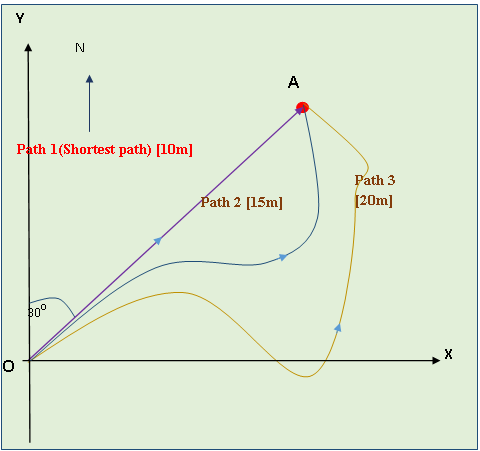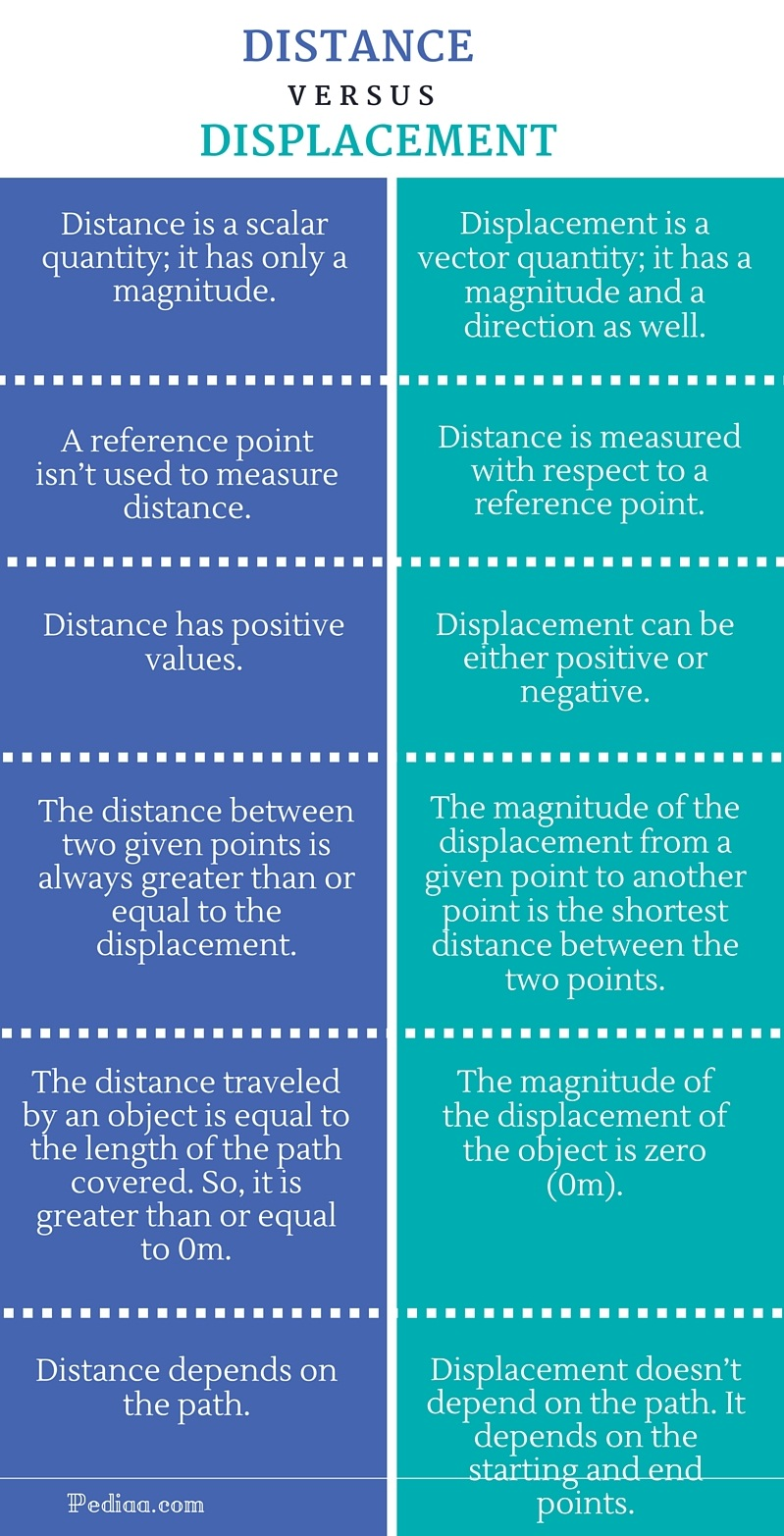# Difference Between Distance and Displacement

## Main Difference – Distance vs Displacement

Distance and displacement are two terms used to describe a path between two given points. There are many paths one can take to reach a given point from another given point. In any case, there is a certain path which is the shortest path between the two given points. Distance and displacement refer to the motion of an object moving from one point to another or the position of a given point relative to another point. Literally, both “distance” and “displacement” give the same idea. But in physics, “distance” and “displacement” cannot be used interchangeably. They have specific and different meanings. The main difference between distance and displacement is that distance is a scalar quantity whereas displacement is a vector quantity.

## What is Distance

Distance is a scalar quantity. Therefore, it only has a magnitude. There are an infinite number of paths to reach a given point from another given point. The following graph shows three such paths between O and A. All three paths start from point O and end at point A. But path 1 has the shortest possible distance between point O and A. The shortest path between any two given points is a straight line segment. The distance of any other path is longer than the path 1 and depends on the path. In the given graph, the distance of the path 2 is greater than that of the path 1. But it is less than the distance of path 3.

Ex:

Distance from O to A via path 1 is 10m.

Distance from O to A via path 2 is 15m.

Distance from O to A via path 3 is 20m.

The distance of a body moved from O to A via path 3 and then came back to the point O via path 2 is 35m. (20m+15m= 35m)

The rate of change of displacement of a moving object is known as the speed.## What is  Displacement

Displacement is a vector quantity. The magnitude of the displacement is equal to the shortest distance between two given points. In the above graph, the shortest path from point O to point A is given by path 1. It is a straight line segment. So the displacement is equal to the length of OA line segment. The magnitude of the displacement of an object moved from O to A is the same via any path regardless of the path. Anyway, it is essential to give the direction whenever we express a displacement because it is a vector quantity.

Ex:

The magnitude of the displacement via path 1 is 10m. The direction is 300 from North to East.

The magnitude of the displacement via path 2 is 10m. The direction is 300 from North to East.

The magnitude of the displacement via path 3 is 10m. The direction is 300 from North to East.

The magnitude of the displacement of a body that moved from O to A via path 3 and then came back to the point O via path 2 is zero (10 m-10 m= 0 m)

The rate of change of displacement of a moving object is known as the velocity.

## Difference Between Distance and Displacement

### Nature of the quantity:

Distance: Distance is a scalar quantity; it has only a magnitude.

Displacement: Displacement is a vector quantity; it has a magnitude and a direction as well.

### SI Unit:

Distance: Distance can be measured in Meter (m).

Displacement: Displacement can be measured in Meter (m).

### Units:

Distance: Distance can be measured in mm, cm, m, km, miles, light years, etc.

Displacement: Displacement can be measured in mm, cm, m, km, miles, light years, etc.

### Reference Point:

Distance: A reference point isn’t used to measure distance.

Displacement: Distance is measured with respect to a reference point.

### Sign:

Distance: Distance has positive values.

Displacement: Displacement can be either positive or negative.

### Other Properties:

Distance: The distance between two given points is always greater than or equal to the displacement.

Displacement: The magnitude of the displacement from a given point to another point is the shortest distance between the two points.

### Distance and displacement of an object that returned to its starting point:

Distance: The distance traveled by an object is equal to the length of the path covered. So, it is greater than or equal to 0m.

Displacement: The magnitude of the displacement of the object is zero (0m).

### Dependence on the path

Distance: Distance depends on the path.

Displacement: Displacement doesn’t depend on the path. It depends on the starting and end points.